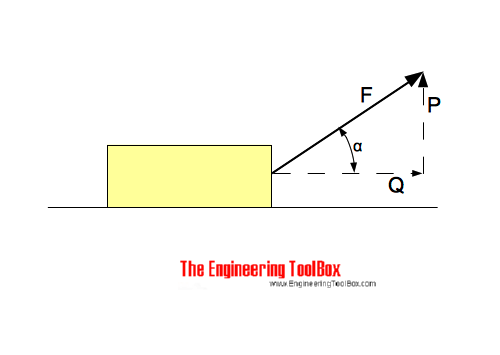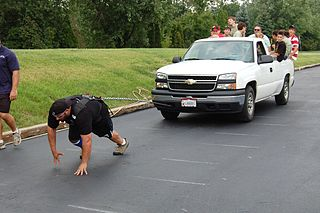Engineering ToolBox - Resources, Tools and Basic Information for Engineering and Design of Technical Applications!

# Forces acting on Body Moving in a Horizontal Plane

## The forces acting on bodies moved in horizontal planes.The force pushing - or pulling - a body along a horizontal surface can be expressed as

Q = F cos α                          (1)

where

Q = force moving the body in horizontal direction (N, lb)

α = angle between force F and horizontal plane

The force acting in vertical direction can be calculated as:

P = (F2 - Q2)1/2                       (2)

where

P = force acting in vertical direction (N, lb)

### Example - Strong Man Pulling a TruckBy stu_spivack [CC-BY-SA-2.0], via Wikimedia Commons

A "strong man" is pulling a truck by exerting a force of 1000 N. The force is acting 30o to the horizontal plane.

The horizontal force on the truck can be calculated as

Q = (1000 N) cos(30o)

= 866 N

= 0.87 kN

The vertical force "lifting" truck can be calculated as

P = ((1000 N)2 - (866 N)2)1/2

= 500 N

= 0.5 kN

### Horizontal and Vertical Force Calculator

The calculator below can used to calculate the horizontal and vertical forces:

F - force acting on the body (N, lb)

α - the angle between the force and the horizontal plane (degrees)

## Related Topics

• Mechanics - Forces, acceleration, displacement, vectors, motion, momentum, energy of objects and more.

## Engineering ToolBox - SketchUp Extension - Online 3D modeling!

Add standard and customized parametric components - like flange beams, lumbers, piping, stairs and more - to your Sketchup model with the Engineering ToolBox - SketchUp Extension - enabled for use with the amazing, fun and free SketchUp Make and SketchUp Pro .Add the Engineering ToolBox extension to your SketchUp from the SketchUp Pro Sketchup Extension Warehouse!

Translate
About the Engineering ToolBox!

## Privacy

We don't collect information from our users. Only emails and answers are saved in our archive. Cookies are only used in the browser to improve user experience.

Some of our calculators and applications let you save application data to your local computer. These applications will - due to browser restrictions - send data between your browser and our server. We don't save this data.

## Citation

• Engineering ToolBox, (2014). Forces acting on Body Moving in a Horizontal Plane. [online] Available at: https://www.engineeringtoolbox.com/force-body-horizontal-move-d_1907.html [Accessed Day Mo. Year].

Modify access date.

. .

#### Scientific Online Calculator9 1